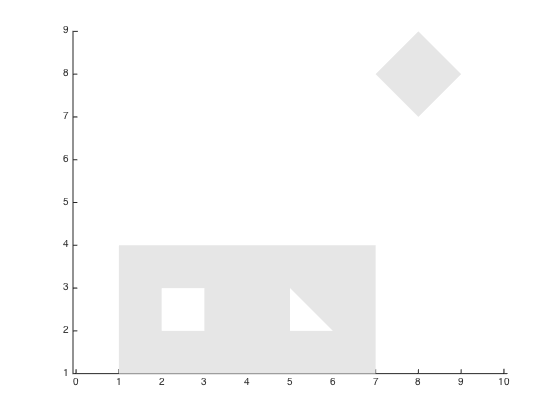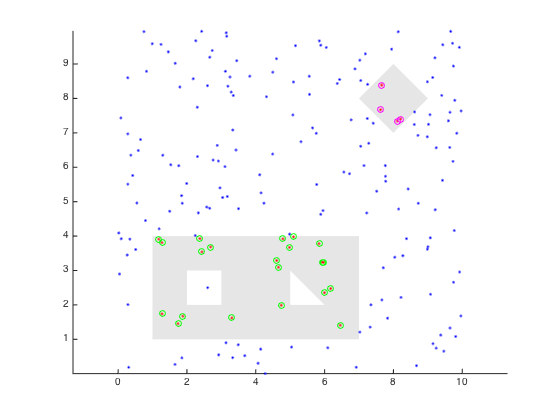## inpolygons

version 1.1.0.1 (21 KB) by
Finds points inside multiple polygons, holes possible.

Updated 23 Sep 2021

From GitHub

## inpolygons.m Documentation

This function checks whether a point or points is inside one or more polygons. The polygons can have holes in them. When dealing with a large number of points and/or polygons, this can be faster that inpolygon.m.

### Syntax

``````in = inpolygons(x,y,xv,yv)
[in, index] = inpolygons(x,y,xv,yv)
``````

See function help for description of input and output variables.

### Example

We'll start with two polygons: a rectangle with square- and triangle-shaped holes, and a solid diamond:

```xv = [1 1 7 7 1 NaN 2 3 3 2 2 NaN 5 6 5 5 NaN 7 8 9 8 7];
yv = [1 4 4 1 1 NaN 2 2 3 3 2 NaN 2 2 3 2 NaN 8 9 8 7 8];

[f, v] = poly2fv(xv, yv);

patch('Faces', f, 'Vertices', v, ...
'FaceColor', [.9 .9 .9], ...
'EdgeColor', 'none');```Now, we scatter a couple hundred random points, and test which polygons they fall into.

```x = 10 * rand(200,1);
y = 10 * rand(200,1);

[in, index] = inpolygons(x, y, xv, yv);
index = cell2mat(index);  % No overlapping polygons allows this.

plot(x(in), y(in), 'r.', x(~in), y(~in), 'b.');
plot(x(index==1), y(index==1), 'go', x(index==2), y(index==2), 'mo');```### Cite As

Kelly Kearney (2021). inpolygons (https://github.com/kakearney/inpolygons-pkg), GitHub. Retrieved .

##### MATLAB Release Compatibility
Created with R14SP1
Compatible with any release
##### Platform Compatibility
Windows macOS Linux